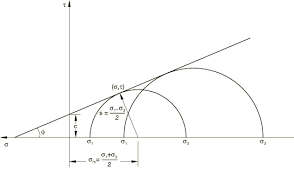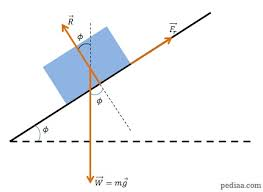## How to Calculate and Solve for Mohr – Coulomb Criterion | Rock MechanicsThe image above represents mohr – coulomb criterion.

To compute for mohr – coulomb criterion, three essential parameters are needed and these parameters are Cohesion (|τo), Co-efficient of Friction (μ) and Normal Stress (σn).

The formula for calculating the mohr – coulomb criterion:

|τ| = τo + μσn

Where;

|τ = Mohr – Coulomb Criterion
o = Cohesion
μ = Co-efficient of Friction
σn = Normal Stress

Let’s solve an example;
Find the mohr – coulomb criterion when the cohesion is 7, the co-efficient of friction is 30 and the normal stress is 28.

This implies that;

o = Cohesion = 7
μ = Co-efficient of Friction = 30
σn = Normal Stress = 28

|τ| = τo + μσn
|τ| = 7 + 30(28)
|τ| = 7 + 840
|τ| = 847

Therefore, the mohr – coulomb criterion is 847.

Calculating the Cohesion when the Mohr – Coulomb Criterion, the Co-efficient of Friction and the Normal Stress is Given.

o = |τ|  – μσn

Where;

o = Cohesion
|τ = Mohr – Coulomb Criterion
μ = Co-efficient of Friction
σn = Normal Stress

Let’s solve an example;
Find the cohesion when the mohr – coulomb criterion is 44, the co-efficient of friction is 10 and the normal stress is 4.

This implies that;

|τ = Mohr – Coulomb Criterion = 44
μ = Co-efficient of Friction = 10
σn = Normal Stress = 4

o = |τ|  – μσn
o = 44  – 10(4)
o = 44  – 40
o = 4

Therefore, the cohesion is 4.

## How to Calculate and Solve for Friction Angle | Rock MechanicsThe image above represents friction angle.

To compute for friction angle, two essential parameters are needed and these parameters are Uniaxial Compressive Strength (σc) and cohesion (c).

The formula for calculating the friction angle:

φ = 2tan-1(σc – 2c / σc + 2c)

Where;

φ = Friction Angle
σc = Uniaxial Compressive Strength
c = Cohesion

Let’s solve an example;
Find the friction angle when the uniaxial compressive strength is 11 and the cohesion is 22.

This implies that;

σc = Uniaxial Compressive Strength = 11
c = Cohesion = 22

φ = 2tan-1(σc – 2c / σc + 2c)
φ = 2tan-1(11 – 2(22) / 11 + 2(22))
φ = 2tan-1(11 – 44 / 11 + 44)
φ = 2tan-1(-33 / 55)
φ = 2tan-1(-0.6)
φ = 2(-30.96°)
φ = -61.927

Therefore,  the friction angle is -61.927.